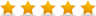Best Python Training Institute in Greater Noida4.9 out of 5 based on 4132 Students ratings.
Course Summary

Python programming is very interesting subject compare to other programming language. Python is not dependent to other language or other program. If you want to become python developer or python expert then must have the practical as well as knowledge on be half of real scenario. Python demand is every where in this world. Python is very secure knowledge because python has very strong encryption as well as decryption. Actually cryptography is divided in two parts first is encryption and second is decryption. We must have the skills to clear the international exam, if we are planning to prepare for the interview then need to prepare the questions for the same. Python has many version also time to time they are launching new version from the international exam.

If will discuss about the job opening in india then there are good jobs opening in all part of the country as well as abroad too. Python actually need to prepare from the overview of the python topics, once this topics will be over then can plan for the companies using python and also about the syntax. Once this module will be over then can plan for different applications where python is used. If this module will be over then need to move to the python scripts on unix and windows. If will complete this topics then can plan for the values, types and variable using python. Once will be over then can plan for the operands and expressions, after this topics can plan for the loops, after the loops can easily plan for the command line arguments, once this arguments will be over then need to learn writing to the screen module. After this theory also can plan for the lab. Lab like crating “Hello World” code, then can prepare for variables, after the variables then can plan for demonstrating conditional statements then can plan for the demonstrating loops about the python also can plan for the machine learning too.

If will discuss about the compatibility then python has compatibility with different subjects. If will discuss about the certification exam on behalf of international exam then can prepare for PCEP(Certified entry level python programmer) means you can familiar with all the universal programming concept in computers, we must have the idea about the data types, containers, functions, conditions, loops, language syntax with semantics and must have the idea about the compilation part as well as the running part. Other certification will be PCAP also called Certified Associate in Python programming.

Course Outline

Python Programming sylPracticalus or Outline for Greater Noida Location

First Week, Assignment 1 => Introduction to Python and Computer Programming
Assignment, Activity & Practical1 => Python => a tool, not a reptile
Assignment, Activity & Practical2 => There is more than one Python
Assignment, Activity & Practical3 => Let's start our Python adventure

Second Week, Assignment 2 => Data Types, Variables, Basic Input=>Output Operations, Basic Operators
Assignment, Activity & Practical1 => Your first program
Assignment, Activity & Practical2 => Python literals
Assignment, Activity & Practical3 => Operators => data manipulation tools
Assignment, Activity & Practical4 => Variables => data=>shaped boxes
Assignment, Activity & Practical5 => How to talk to computer?

Third Week, Assignment 3 => Boolean Values, Conditional Execution, Loops, Lists and List Processing, Logical and Bitwise Operations
Assignment, Activity & Practical1 => Making decisions in Python
Assignment, Activity & Practical2 => Python's loops
Assignment, Activity & Practical3 => Logic and bit operations in Python
Assignment, Activity & Practical4 => Lists => collections of data
Assignment, Activity & Practical5 => Sorting simple lists => the bubble sort algorithm
Assignment, Activity & Practical6 => Lists => some more details
Assignment, Activity & Practical7 => Lists in advanced applications

Fourth Week, Assignment 4 => Functions, Tuples, Dictionaries, and Data Processing
Assignment, Activity & Practical1 => Writing functions in Python
Assignment, Activity & Practical2 => How functions communicate with their environment?
Assignment, Activity & Practical3 => Returning a result from a function
Assignment, Activity & Practical4 => Scopes in Python
Assignment, Activity & Practical5 => Let's make some fun... sorry, functions
Assignment, Activity & Practical6 =>Tuples and dictionaries

Fifth Week, Assignment 5 => Assignments, Packages, String and List Methods, and Exceptions
Assignment, Activity & Practical1 => Using Assignments
Assignment, Activity & Practical2 => Some useful Assignments
Assignment, Activity & Practical3 => What is package?
Assignment, Activity & Practical4 => Errors => the programmer's daily bread
Assignment, Activity & Practical5 => The anatomy of exception
Assignment, Activity & Practical6 => Some of the most useful exceptions
Assignment, Activity & Practical7 => Characters and strings vs. computers
Assignment, Activity & Practical8 => Python's nature of strings
Assignment, Activity & Practical9 => String methods
Assignment, Activity & Practical10 => Strings in action
Assignment, Activity & Practical11 => Four simple programs

Sixth Week, Assignment 6 => The Object=>Oriented Approach: Classes, Methods, Objects, and the Standard Objective Features; Exception Handling, and Working with Files
Assignment, Activity & Practical1 => Basic concepts of object programming
Assignment, Activity & Practical2 => A short journey from procedural to object approach
Assignment, Activity & Practical3 => Properties
Assignment, Activity & Practical4 => Methods
Assignment, Activity & Practical5 => Inheritance => one of object programming foundations
Assignment, Activity & Practical6 => Exceptions once again
Assignment, Activity & Practical7 => Generators and closures
Assignment, Activity & Practical8 => Processing files
Assignment, Activity & Practical9 => Working with real files

Course Features

• Duration : 40 Hours
• Lectures : 20
• Quiz : 10
• Students : 15

You may like